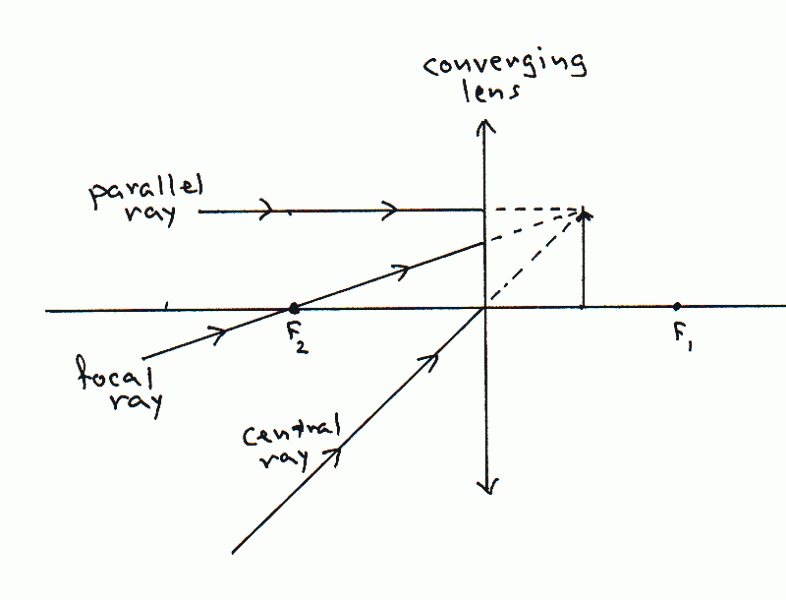# Hand drawn ray tracing through an optical system

• optotinker
If the object is at infinity as I stated, wouldn't all lines be parallel to the optical axis?If all your rays from an object at infinity were parallel to the optical axis then you would not be able to form an image at all.f

#### optotinker

Hi,

I am familiar with drawing rays through a lens. But when a few lenses are put together, things become confusing to me.

For example, if a first positive thin lens at 0 forms a real image 10cm away, what would happen when we put a second positive thin lens, say at 5cm along the optical path. Now the virtual image formed by the 1st lens is on the wrong side of the 2nd lens. Is there still a way to graph this with just pencil and ruler alone?

Any reference would be appreciated.

Tinker

Hand drawn analysis is great for building intuition, not for doing real quantitative work.

I recall seeing some old textbook that describes how to do that.

Hand drawing is great for intuition, but when things get to complex it simply does not work anymore. Also, with hand drawing it is very difficult to get precise results, let alone optimize a setup.

For your particular example, it does not really matter on which side of the second lens the virtual image is. The same basic rules apply:
A ray that goes through the center of the lens is just a straight line.
A ray that goes through the focal point comes out parallel to the optical axis
A ray that is parallel to the optical axis goes through the focal point.

Just draw these 3 lines (principal rays) from the virtual image to the second lens

I find it helps to use a different color for the rays of the second lens. With more and more lines things become more and more confusing.

If you search for "geometric optics" you can find a lot more information, including some old books.

The difficulty I have is that these three rays from the first lens generally come out at odd angles, neither going through focal point of the 2nd lens or being parallel to the optical axis.

No. You draw new ones from the virtual source. Actually, you can reuse the one that is parallel to the optical axis.

I am still not clear.

Consider the case with object at infinity, the first lens has f=10cm, the second lens has f=5 and placed at 5cm down the optical axis from the 1st lens, how do I find the combined focus position of these two lenses?

You draw two parallel lines, NOT parallel to the optical axis. One through the center of the first lens (this one goes straight), the second through the focal point (this one becomes parallel to the optical axis). They will cross at the focal distance behind the lens, forming a (virtual) image.

I let you work out what the second lens does.

If the object is at infinity as I stated, wouldn't all lines be parallel to the optical axis?

I know what the first or second lenses do individually. I also know how to draw if the 2nd lens further away from the 1st lens by a distance larger than the focal length.

But I don't know how to DRAW if the virtual image is to the right of the 2nd lens.

With all incident lines parallel to the optical axis the diagram does not work in this case.

If all your rays from an object at infinity were parallel to the optical axis then you would not be able to form an image at all.

So rays from the "tip" of the object have to come at a (very small) angle. Drawing very small angles is not practical, so one just exaggerates. That the object is at infinity is taken into account by the fact that the rays are parallel, i.e. all are at the same angle.

If the object was at a finite distance from the lens, then rays from the same point on the object would hit different parts of the lens at different angles.

Just draw the diagram backwards, inverting source and image.

You know that the image plane is 2.5 cm from the second lens...

Now the virtual image formed by the 1st lens is on the wrong side of the 2nd lens. Is there still a way to graph this with just pencil and ruler alone?

It's easier to see what happens if you draw a diagram that omits the original object and the first lens, and shows only the rays arriving at the second lens, headed towards the image produced by the first lens. Of course, most of the rays never actually arrive at that first image, because the second lens deviates them. We call this a virtual object for the second lens. A Google search on "virtual object" will probably turn up some more information.

Just like with the ray diagrams you've probably seen already, there are three principal rays, with similar rules:
1. The parallel ray, which comes in parallel to the axis and goes out through the first focal point, F1.
2. The focal ray, which comes in through the second focal point, F2, and goes out parallel to the axis.
3. The central ray, which goes through the center of the lens undeviated.
In the example shown here, I've drawn the incoming principal rays for a virtual object. Try following the rules above to draw the outgoing rays.Last edited: